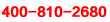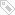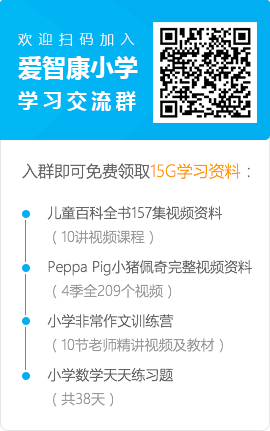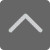# 北京小学一年级数学上册期末考试模拟题

2019-05-09 08:12:00 　来源：网络整理

北京小学一年级数学上册期末考试模拟题同学们，我们学习需要及时复习，及时完成老师布置得作业，还要多做课后习题进行巩固。下面就是小编为大家带来的北京小学一年级数学上册期末考试模拟题，希望可以帮助到大家。

想要了解【小学数学试题】的相关资料，请点击加入【爱智康小学交流福利群】，并直接向管理员“小康康”索取！爱智康小学交流福利群会不定期免费发放学习资料，小学政策等相关消息，请持续关注！

一、口算。

5+2=答案

9+3=答案

6+5=答案

8+4=答案

9-5=答案

10-6=答案

3+6=答案

6+4=答案

18-8=答案

7-7=答案

7+8=答案

9+6=答案

6+7=答案

10-7=答案

3+5=答案

7+4=答案

8+9=答案

7+7=答案

12-2=答案

9-6=答案

2+9=答案

5+8=答案

7+9=答案

9+4=答案

9+5=答案

1+9=答案

12-10=答案

6+10=答案

6+8=答案

6+9=答案

二、填空。

1. 1个十和5个一组成的数是( )。

2. 17里面有( )个十和( )个一。

3. 6个一和1个十组成的数是( )。

4. 个位是3，十位是1，这个数是( )。

5. 10比8多( )，3比4少( )。

6. 15比17少( )，18比10多( )。

7. ☆□○△□□○○○☆?

(1) 一共有( )个图形。

(2) ☆有( )个，□有( )个， 有( )个，○有( )个，△有( )个。

(3) 把右边的8个图形圈起来。

(4) 从右往左数第6个图形是( )。

8. 在□里填数。

(1) 0、6、15、9、7、2。

□>□>□>□>□>□

(2) 8、1、5、9、11、13、4

□<□<□<□<□<□<□

三、按顺序填空。

(1)_____ _____ 6 _____ _____ 9 _____。

(2)14_____ _____ 17 _____ _____ _____ 。

(3)19_____ _____ _____ 15_____ _____ 。

六、填空。

(1) 9添上1是( )，19添上1是( )。

(2) 16里面有( )个十和( )个一。

(3) 1个十是( )个一。

(4) 大于11又小于13的数是( )。

(5) 一个两位数，从右边起第一位是( )位，第二位是( )位。

(6) 12里面一共有( )个一。

四、在○里填上“>”、“<”或“=”。

8+5○14 　　14-4○10

7+8○8+7 　　5+9○6+7

9○4+6　　 10○10-2

五、在○里填上“+”或“-”。

3○6=9

8○5=3

12○2=10

10○4=14

18○10=8

7○7=0

10○9=19

6○6=12

5○4=9

7○2=5

7○9=16

9○1=8

六、看谁算得又对又快。

9+2=答案

8+3=答案

7+4=答案

6+7=答案

7+5=答案

4+6=答案

9+8=答案

6+5=答案

5+4=答案

10-3=答案

9-8=答案

7-6=答案

8-5=答案

9-9=答案

6+6=答案

10+5=答案

6+8=答案

15-10=答案

13+2=答案

4+10=答案

17-7=答案

4+9=答案

10-9=答案

9-7=答案

3+1+4=答案

9-7-1=答案

7+0+1=答案

2+7-3=答案

10-6+5=答案

9+9-10=答案

8-4+3=答案

8-6+7=答案

10-9+6=答案

5+8-3=答案

6+6-2=答案

7-7+5=答案

七、列式计算。

1. 两个加数都是8，和是多少?

2. 减数是7，被减数是17，差是多少?

3. 甲、乙两个数的和是15，甲数是10，乙数是几?

八、写出20以内进位加法中，得数是11的八个不同算式。

_____________________________________

_____________________________________

九、列式计算

①一个加数是9，另一个加数是5，和是多少?

_______________________

②被减数是10，减数是4，差是多少?

_______________________

小编推荐：

以上就是小编特意为大家整理的北京小学一年级数学上册期末考试模拟题希望对有需要的同学提供帮助，大家如果在学习中有什么疑问，欢迎拨打爱智康免费电话：!那里有专业的老师为大家解答。

### 2019年精品学习包：

•### 小学语|数|英

历年试卷、北京30所校练习题、名著|重点校巡礼|等100讲视频、纸质书籍领取

•### 初中九大学科

历年真题、期末|期中|志愿填报|重点校巡礼|等100讲视频、纸质书籍领取

•### 高中九大学科

历年真题、期末|期中|志愿填报|重点校巡礼|等100讲视频、纸质书籍领取

•### 更新中

学习包内容持续更新中......标签：• 全国课程在线咨询
• 咨询热线：4000-121-121
• 扫描注册有礼

• 让学习更有效